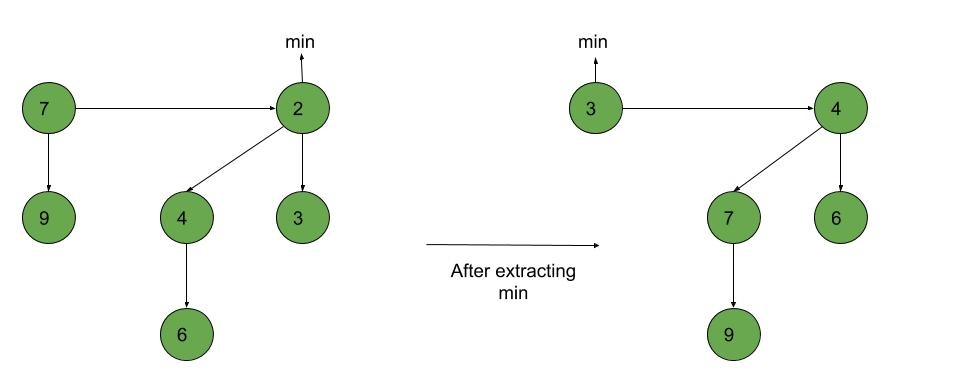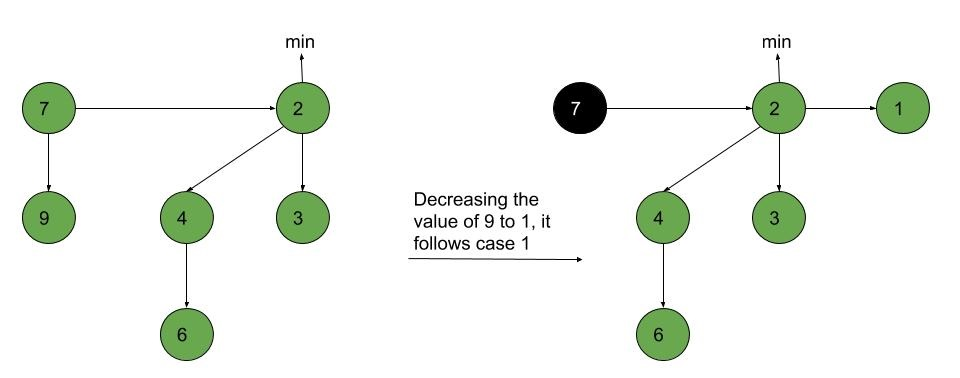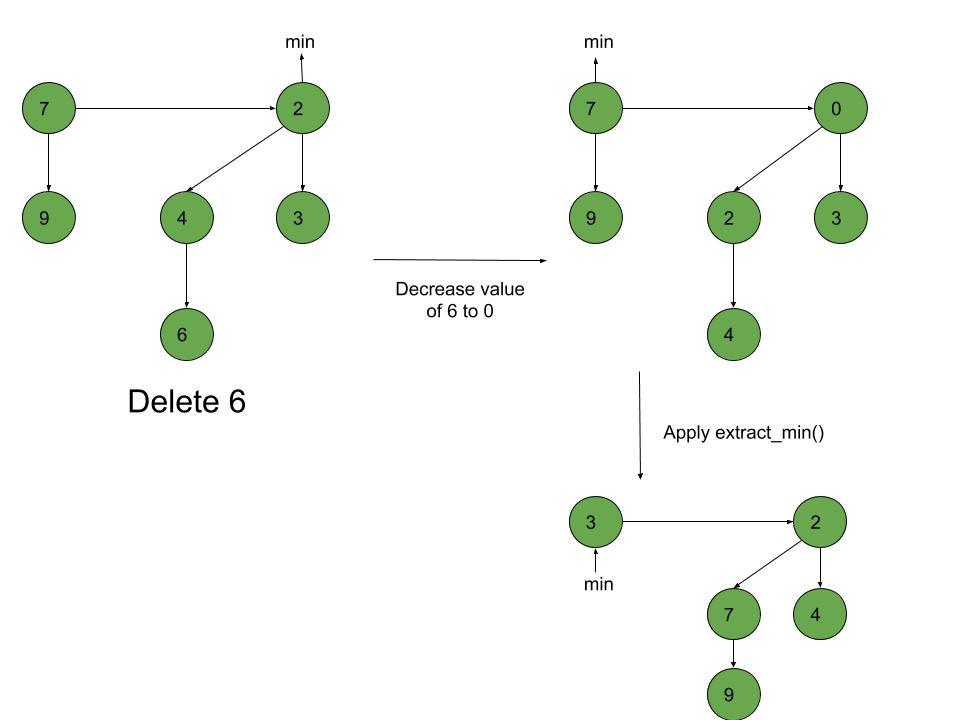Fibonacci Heap – Deletion, Extract min and Decrease key

• Difficulty Level : Hard
• Last Updated : 24 Nov, 2021

In the last post, we discussed the Insertion and Union of Fibonacci Heaps. In this post, we will discuss Extract_min(), Decrease_key() and Deletion() operations on Fibonacci heap.

Extract_min(): We create a function for deleting the minimum node and setting the min pointer to the minimum value in the remaining heap. The following algorithm is followed:

1. Delete the min node.
2. Set head to the next min node and add all the trees of the deleted node in the root list.
3. Create an array of degree pointers of the size of the deleted node.
4. Set degree pointer to the current node.
5. Move to the next node.
• If degrees are different then set degree pointer to next node.
• If degrees are the same then join the Fibonacci trees by union operation.
6. Repeat steps 4 and 5 until the heap is completed.

Example:Decrease_key(): To decrease the value of any element in the heap, we follow the following algorithm:

• Decrease the value of the node ‘x’ to the new chosen value.
• CASE 1) If min-heap property is not violated,
• Update min pointer if necessary.
• CASE 2) If min-heap property is violated and parent of ‘x’ is unmarked,
• Cut off the link between ‘x’ and its parent.
• Mark the parent of ‘x’.
• Add tree rooted at ‘x’ to the root list and update min pointer if necessary.
• CASE 3)If min-heap property is violated and parent of ‘x’ is marked,
• Cut off the link between ‘x’ and its parent p[x].
• Add ‘x’ to the root list, updating min pointer if necessary.
• Cut off link between p[x] and p[p[x]].
• Add p[x] to the root list, updating min pointer if necessary.
• If p[p[x]] is unmarked, mark it.
• Else, cut off p[p[x]] and repeat steps 4.2 to 4.5, taking p[p[x]] as ‘x’.

Example:Deletion(): To delete any element in a Fibonacci heap, the following algorithm is followed:

1. Decrease the value of the node to be deleted ‘x’ to a minimum by Decrease_key() function.
2. By using min-heap property, heapify the heap containing ‘x’, bringing ‘x’ to the root list.
3. Apply Extract_min() algorithm to the Fibonacci heap.

Example:Following is a program to demonstrate Extract min(), Deletion() and Decrease key() operations on a Fibonacci Heap:

C++

 // C++ program to demonstrate Extract min, Deletion()// and Decrease key() operations in a fibonacci heap#include #include #include #include using namespace std;  // Creating a structure to represent a node in the heapstruct node {    node* parent; // Parent pointer    node* child; // Child pointer    node* left; // Pointer to the node on the left    node* right; // Pointer to the node on the right    int key; // Value of the node    int degree; // Degree of the node    char mark; // Black or white mark of the node    char c; // Flag for assisting in the Find node function};  // Creating min pointer as "mini"struct node* mini = NULL;  // Declare an integer for number of nodes in the heapint no_of_nodes = 0;  // Function to insert a node in heapvoid insertion(int val){    struct node* new_node = new node();    new_node->key = val;    new_node->degree = 0;    new_node->mark = 'W';    new_node->c = 'N';    new_node->parent = NULL;    new_node->child = NULL;    new_node->left = new_node;    new_node->right = new_node;    if (mini != NULL) {        (mini->left)->right = new_node;        new_node->right = mini;        new_node->left = mini->left;        mini->left = new_node;        if (new_node->key < mini->key)            mini = new_node;    }    else {        mini = new_node;    }    no_of_nodes++;}// Linking the heap nodes in parent child relationshipvoid Fibonnaci_link(struct node* ptr2, struct node* ptr1){    (ptr2->left)->right = ptr2->right;    (ptr2->right)->left = ptr2->left;    if (ptr1->right == ptr1)        mini = ptr1;    ptr2->left = ptr2;    ptr2->right = ptr2;    ptr2->parent = ptr1;    if (ptr1->child == NULL)        ptr1->child = ptr2;    ptr2->right = ptr1->child;    ptr2->left = (ptr1->child)->left;    ((ptr1->child)->left)->right = ptr2;    (ptr1->child)->left = ptr2;    if (ptr2->key < (ptr1->child)->key)        ptr1->child = ptr2;    ptr1->degree++;}// Consolidating the heapvoid Consolidate(){    int temp1;    float temp2 = (log(no_of_nodes)) / (log(2));    int temp3 = temp2;    struct node* arr[temp3+1];    for (int i = 0; i <= temp3; i++)        arr[i] = NULL;    node* ptr1 = mini;    node* ptr2;    node* ptr3;    node* ptr4 = ptr1;    do {        ptr4 = ptr4->right;        temp1 = ptr1->degree;        while (arr[temp1] != NULL) {            ptr2 = arr[temp1];            if (ptr1->key > ptr2->key) {                ptr3 = ptr1;                ptr1 = ptr2;                ptr2 = ptr3;            }            if (ptr2 == mini)                mini = ptr1;            Fibonnaci_link(ptr2, ptr1);            if (ptr1->right == ptr1)                mini = ptr1;            arr[temp1] = NULL;            temp1++;        }        arr[temp1] = ptr1;        ptr1 = ptr1->right;    } while (ptr1 != mini);    mini = NULL;    for (int j = 0; j <= temp3; j++) {        if (arr[j] != NULL) {            arr[j]->left = arr[j];            arr[j]->right = arr[j];            if (mini != NULL) {                (mini->left)->right = arr[j];                arr[j]->right = mini;                arr[j]->left = mini->left;                mini->left = arr[j];                if (arr[j]->key < mini->key)                    mini = arr[j];            }            else {                mini = arr[j];            }            if (mini == NULL)                mini = arr[j];            else if (arr[j]->key < mini->key)                mini = arr[j];        }    }}  // Function to extract minimum node in the heapvoid Extract_min(){    if (mini == NULL)        cout << "The heap is empty" << endl;    else {        node* temp = mini;        node* pntr;        pntr = temp;        node* x = NULL;        if (temp->child != NULL) {              x = temp->child;            do {                pntr = x->right;                (mini->left)->right = x;                x->right = mini;                x->left = mini->left;                mini->left = x;                if (x->key < mini->key)                    mini = x;                x->parent = NULL;                x = pntr;            } while (pntr != temp->child);        }        (temp->left)->right = temp->right;        (temp->right)->left = temp->left;        mini = temp->right;        if (temp == temp->right && temp->child == NULL)            mini = NULL;        else {            mini = temp->right;            Consolidate();        }        no_of_nodes--;    }}  // Cutting a node in the heap to be placed in the root listvoid Cut(struct node* found, struct node* temp){    if (found == found->right)        temp->child = NULL;      (found->left)->right = found->right;    (found->right)->left = found->left;    if (found == temp->child)        temp->child = found->right;      temp->degree = temp->degree - 1;    found->right = found;    found->left = found;    (mini->left)->right = found;    found->right = mini;    found->left = mini->left;    mini->left = found;    found->parent = NULL;    found->mark = 'B';}  // Recursive cascade cutting functionvoid Cascase_cut(struct node* temp){    node* ptr5 = temp->parent;    if (ptr5 != NULL) {        if (temp->mark == 'W') {            temp->mark = 'B';        }        else {            Cut(temp, ptr5);            Cascase_cut(ptr5);        }    }}  // Function to decrease the value of a node in the heapvoid Decrease_key(struct node* found, int val){    if (mini == NULL)        cout << "The Heap is Empty" << endl;      if (found == NULL)        cout << "Node not found in the Heap" << endl;      found->key = val;      struct node* temp = found->parent;    if (temp != NULL && found->key < temp->key) {        Cut(found, temp);        Cascase_cut(temp);    }    if (found->key < mini->key)        mini = found;}  // Function to find the given nodevoid Find(struct node* mini, int old_val, int val){    struct node* found = NULL;    node* temp5 = mini;    temp5->c = 'Y';    node* found_ptr = NULL;    if (temp5->key == old_val) {        found_ptr = temp5;        temp5->c = 'N';        found = found_ptr;        Decrease_key(found, val);    }    if (found_ptr == NULL) {        if (temp5->child != NULL)            Find(temp5->child, old_val, val);        if ((temp5->right)->c != 'Y')            Find(temp5->right, old_val, val);    }    temp5->c = 'N';    found = found_ptr;}  // Deleting a node from the heapvoid Deletion(int val){    if (mini == NULL)        cout << "The heap is empty" << endl;    else {          // Decreasing the value of the node to 0        Find(mini, val, 0);          // Calling Extract_min function to        // delete minimum value node, which is 0        Extract_min();        cout << "Key Deleted" << endl;    }}  // Function to display the heapvoid display(){    node* ptr = mini;    if (ptr == NULL)        cout << "The Heap is Empty" << endl;      else {        cout << "The root nodes of Heap are: " << endl;        do {            cout << ptr->key;            ptr = ptr->right;            if (ptr != mini) {                cout << "-->";            }        } while (ptr != mini && ptr->right != NULL);        cout << endl             << "The heap has " << no_of_nodes << " nodes" << endl             << endl;    }}  // Driver codeint main(){    // We will create a heap and insert 3 nodes into it    cout << "Creating an initial heap" << endl;    insertion(5);    insertion(2);    insertion(8);      // Now we will display the root list of the heap    display();      // Now we will extract the minimum value node from the heap    cout << "Extracting min" << endl;    Extract_min();    display();      // Now we will decrease the value of node '8' to '7'    cout << "Decrease value of 8 to 7" << endl;    Find(mini, 8, 7);    display();      // Now we will delete the node '7'    cout << "Delete the node 7" << endl;    Deletion(7);    display();      return 0;}

Python3

 # Python3 program to demonstrate Extract min, Deletion()# and Decrease key() operations in a fibonacci heapimport math  # Creating a class to represent a node in the heapclass node:    def __init__(self):        parent=None # Parent pointer        child=None # Child pointer        left=None # Pointer to the node on the left        right=None # Pointer to the node on the right        key=-1 # Value of the node        degree=-1 # Degree of the node        mark='' # Black or white mark of the node        c='' # Flag for assisting in the Find node function  # Creating min pointer as "mini"mini = None  # Declare an integer for number of nodes in the heapno_of_nodes = 0  # Function to insert a node in heapdef insertion(val):    global mini,no_of_nodes      new_node = node()    new_node.key = val    new_node.degree = 0    new_node.mark = 'W'    new_node.c = 'N'    new_node.parent = None    new_node.child = None    new_node.left = new_node    new_node.right = new_node    if (mini != None):        mini.left.right = new_node        new_node.right = mini        new_node.left = mini.left        mini.left = new_node        if (new_node.key < mini.key):            mini = new_node    else:        mini = new_node    no_of_nodes+=1  #  Linking the heap nodes in parent child relationshipdef Fibonnaci_link(ptr2, ptr1):    ptr2.left.right = ptr2.right    ptr2.right.left = ptr2.left    if (ptr1.right == ptr1):        mini = ptr1    ptr2.left = ptr2    ptr2.right = ptr2    ptr2.parent = ptr1    if (ptr1.child == None):        ptr1.child = ptr2    ptr2.right = ptr1.child    ptr2.left = ptr1.child.left    ptr1.child.left.right = ptr2    ptr1.child.left = ptr2    if ptr2.key < ptr1.child.key:        ptr1.child = ptr2    ptr1.degree+=1  # Consolidating the heapdef Consolidate():          global mini    temp2 = math.log2(no_of_nodes)    temp3 = int(temp2)    arr=[None]*(temp3+1)    for i in range(temp3+1):        arr[i] = None    ptr1 = mini    ptr4 = ptr1    while True:        ptr4 = ptr4.right        temp1 = ptr1.degree        while (arr[temp1] != None):            ptr2 = arr[temp1]            if (ptr1.key > ptr2.key):                ptr3 = ptr1                ptr1 = ptr2                ptr2 = ptr3            if (ptr2 == mini):                mini = ptr1            Fibonnaci_link(ptr2, ptr1)            if (ptr1.right == ptr1):                mini = ptr1            arr[temp1] = None            temp1+=1        arr[temp1] = ptr1        ptr1 = ptr1.right        if (ptr1 == mini):            break    mini = None    for j in range(temp3+1):        if (arr[j] != None):            arr[j].left = arr[j]            arr[j].right = arr[j]            if (mini != None) :                mini.left.right = arr[j]                arr[j].right = mini                arr[j].left = mini.left                mini.left = arr[j]                if (arr[j].key < mini.key):                    mini = arr[j]            else:                mini = arr[j]            if mini == None:                mini = arr[j]            elif arr[j].key < mini.key:                mini = arr[j]        # Function to extract minimum node in the heapdef Extract_min():      global mini,no_of_nodes    if mini == None:        print("The heap is empty")    else:        temp = mini        pntr = temp        x = None        if (temp.child != None):              x = temp.child            while(True):                pntr = x.right                mini.left.right = x                x.right = mini                x.left = mini.left                mini.left = x                if x.key < mini.key:                    mini = x                x.parent = None                x = pntr                if (pntr == temp.child):                    break          temp.left.right = temp.right        temp.right.left = temp.left        mini = temp.right        if temp == temp.right and temp.child == None:            mini = None        else:            mini = temp.right            Consolidate()        no_of_nodes-=1      # Cutting a node in the heap to be placed in the root listdef Cut(found, temp):      if (found == found.right):        temp.child = None      found.left.right = found.right    found.right.left = found.left    if (found == temp.child):        temp.child = found.right      temp.degree = temp.degree - 1    found.right = found    found.left = found    mini.left.right = found    found.right = mini    found.left = mini.left    mini.left = found    found.parent = None    found.mark = 'B'  # Recursive cascade cutting functiondef Cascase_cut(temp):      ptr5 = temp.parent    if (ptr5 != None):        if (temp.mark == 'W'):            temp.mark = 'B'        else:            Cut(temp, ptr5)            Cascase_cut(ptr5)  # Function to decrease the value of a node in the heapdef Decrease_key(found, val):      global mini    if (mini == None):        print("The Heap is Empty")      if found == None:        print("Node not found in the Heap")      found.key = val      temp = found.parent    if (temp != None and found.key < temp.key):        Cut(found, temp)        Cascase_cut(temp)      if (found.key < mini.key):        mini = found    # Function to find the given nodedef Find(mini, old_val, val):      found = None    temp5 = mini    temp5.c = 'Y'    found_ptr = None    if (temp5.key == old_val):        found_ptr = temp5        temp5.c = 'N'        found = found_ptr        Decrease_key(found, val)      if (found_ptr == None):        if (temp5.child != None):            Find(temp5.child, old_val, val)        if temp5.right.c != 'Y':            Find(temp5.right, old_val, val)    temp5.c = 'N'    found = found_ptr    # Deleting a node from the heapdef Deletion(val):      if (mini == None):        print("The heap is empty")    else:        # Decreasing the value of the node to 0        Find(mini, val, 0)          # Calling Extract_min function to        # delete minimum value node, which is 0        Extract_min()        print("Key Deleted")    # Function to display the heapdef display():    ptr = mini    if (ptr == None):        print("The Heap is Empty")      else:        print("The root nodes of Heap are: ")        while(True):            print(ptr.key,end='')            ptr = ptr.right            if (ptr != mini):                print("-->",end='')            if not(ptr != mini and ptr.right != None):                break        print()        print("The heap has {} nodes".format(no_of_nodes))  # Driver codeif __name__ == '__main__':      # We will create a heap and insert 3 nodes into it    print("Creating an initial heap")    insertion(5)    insertion(2)    insertion(8)      # Now we will display the root list of the heap    display()      # Now we will extract the minimum value node from the heap    print("Extracting min")    Extract_min()    display()      # Now we will decrease the value of node '8' to '7'    print("Decrease value of 8 to 7")    Find(mini, 8, 7)    display()      print("Now we will delete the node '7'")    print("Delete the node 7")    Deletion(7)    display()  # This code is contributed by Amartya Ghosh
Output:
Creating an initial heap
The root nodes of Heap are:
2-->5-->8
The heap has 3 nodes

Extracting min
The root nodes of Heap are:
5
The heap has 2 nodes

Decrease value of 8 to 7
The root nodes of Heap are:
5
The heap has 2 nodes

Delete the node 7
Key Deleted
The root nodes of Heap are:
5
The heap has 1 nodes

My Personal Notes arrow_drop_up